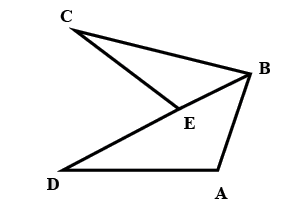Filters
Latest Questions
Mathematics
Inequality and geometric application
If $x$ is a negative integer,then find the solution set of the inequality
$\dfrac{2}{3} + \dfrac{1}{3}(x + 1) > 0$
Mathematics
Inequality and geometric application
How do you solve $\left| -10x-5 \right|=0$?
Mathematics
Inequality and geometric application
How do you rewrite the inequality $\left| {11 - 2x} \right| \geqslant 13$ as a compounded inequality?
Mathematics
Inequality and geometric application
How do you solve two step inequalities?
Mathematic
Inequality and geometric application
How to solve the inequality $\dfrac{-x}{7}+4\ge 3x$ ?
Mathematics
Inequality and geometric application
How do you graph and solve $\left| {4x + 8} \right| \geqslant 20$?
Mathematics
Inequality and geometric application
How do you solve $5x\ge 30$ ?
Mathematics
Inequality and geometric application
Consider the sentence : $x < 5 \\ \\$
Which of the following integers make this open sentence true?
A. 4
B. 5
C. 6
D. None of the above
Mathematics
Inequality and geometric application
A manufacturing company makes two types of teaching aids A and B of mathematics for class XII. Each type of A requires 9 labour hours of fabricating and 1 labour hour for finishing. Each type of B requires 12 labour hours for fabricating and 3 labour hours for finishing. For fabricating and finishing, the maximum labour hours per week are 180 and 30 respectively. The company makes a profit of 80 on each piece of type A and 120 on each piece of type B. How many pieces of type A and type B should be manufactured per week to get a maximum profit? Make it as an LPP and solve graphically. What is the maximum profit per week?

Mathematics
Inequality and geometric application
For a > b > c > 0, the distance between (1, 1) and the point of intersection of the line ax + by + c = 0 and bx + ay + c = 0 is less than $2\sqrt 2$, then
(A). a + b – c > 0
(B). a – b + c < 0
(C). a – b + c > 0
(D). a + b – c < 0
Mathematics
Inequality and geometric application
Given$ED = EC,$
Then $AB + AD > BC$
If the above statement is true, then mention answer as 1, else mention 0 if it is falseMathematics
Inequality and geometric application
A farmer has 10 acres of land to plant wheat and rye. He has to plant at least 7 acres. Each acre of wheat costs ${$}$200 and each acre of rye costs${$}$100 to plant. He has only ${$}$1200 to spend. Moreover, the farmer has to get the planting done in 12 hours and it takes 1 hour to plant an acre of wheat and 2 hours to plant an acre of rye. An acre of wheat yields a profit of${$}$500 and an acre of rye yields a profit of ${$}$300. (Take x and y as the acres of wheat and rye planted respectively). What is the maximum profit that the farmer can make? A.${$}$2500
B. ${$}$2800 C.${$}$3100
D. ${$}\$3200

Prev
1
2